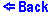Kirchhoff's Rules (Circuit 1)
Increase/decrease the magnitude of the EMFs and the resistors by clicking on the upper/lower part of the object. The values of the resistors range from 1 to 10 ohms. And the values of the EMFs range from -10 to +10 Volts. The ammeters show the currents in the wires, the voltmeters show the voltages across the resistors.
The circuit consists of a loop ACDF with no branches, as a result there is only one current in the whole loop. On each side of the loop there are components: an EMF, an Ammeter which measures the current through that loop, a resistor, and a Voltmeter which measures the potential rise/drop on the resistor. The values for each of the components can be seen either next to or written on the components themselves. It should be made clear that the Ammeters and Voltmeters are positioned in such a way that they will show a positive reading when the current is flowing from top to bottom, although such a selection is made in this circuit it would have been just as correct to pick it's opposite.

Only two rules/formulas are needed to solve the system of equations envolved:

• 1. Kirchhoff's Loop Rule and
• 2. Ohm's Law (i.e. V/I = R,
• where I is the current, V is the voltage, and R is the resistance).

To solve the equation we will need to name our components, we have chosen a system in which each component is named by a letter indicating it's type (I for current, E for the EMF, and R for resistance) and a subletter indicating it's branch (A or C). Thus the current in the leftmost branch would be (by Ohm's Law): I = VA/RA.

We apply the Loop Rule, which says that the sum of the potential rises must equal to the sum of the potential drops i.e.,
EA + I*RA + I*RC = EC;
I = (EC - EA)/(RA + RC);

Therefore, since the resistance, R, and the EMF, E, are known for each side, the currents, I, are calculated by the above method.

The voltage across the resistors is arrived at as follows: (let V be the voltage across the resistor, by Ohm's Law)
V = IR;© Copyright 1997, Sergey Kiselev and Tanya Yanovsky-Kiselev
Last modified: June 20, 1997